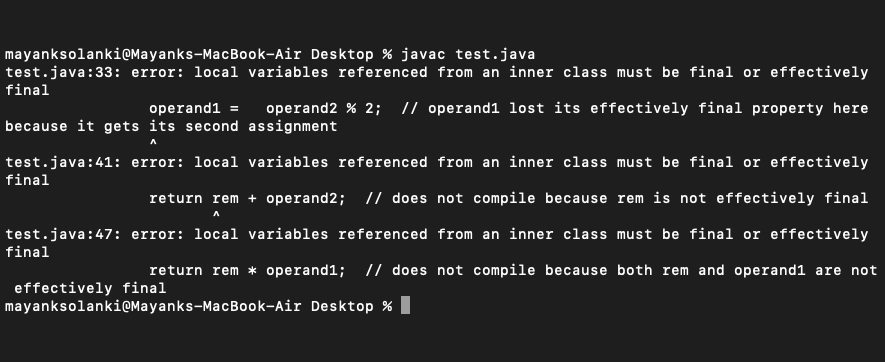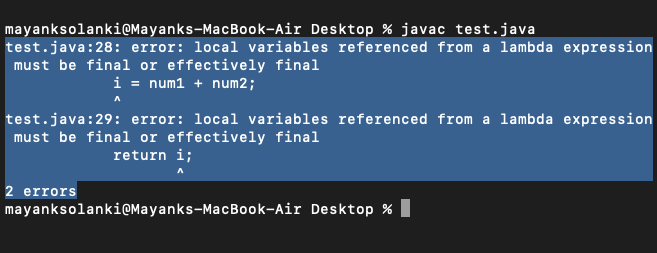# Effectively Final Variable in Java with Examples

• Last Updated : 17 Aug, 2021

A final variable is a variable that is declared with a keyword known as ‘final‘.

Example:

Attention reader! Don’t stop learning now. Get hold of all the important Java Foundation and Collections concepts with the Fundamentals of Java and Java Collections Course at a student-friendly price and become industry ready. To complete your preparation from learning a language to DS Algo and many more,  please refer Complete Interview Preparation Course.

```final int number;
number = 77;```

The Effectively Final variable is a local variable that follows the following properties are listed below as follows:

• Not defined as final
• Assigned to ONLY once.

Any local variable or parameter that’s assigned a worth just one occasion right now(or updated only once). It may not remain effectively final throughout the program. so this suggests that effectively final variable might lose its effectively final property after immediately the time it gets assigned/updated a minimum of another assignment. Additionally, an effectively final variable may be a variable whose value isn’t changed, but it isn’t declared with the ultimate keyword.

```int number;
number = 7;```

Note: Final and Effective Final are similar but just that effective Final variables are not declared with Keyword final.

Example:

## Java

 `// Java Program to Illustrate Effective Final Keyword`` ` `// Importing input output classes``import` `java.io.*;`` ` `// Main class``class` `GFG {`` ` `    ``// Main friver method``    ``public` `static` `void` `main(String[] args)``    ``{`` ` `        ``// Calling the method where custom operands``        ``// are passed as parameters``        ``calculateValue(``124``, ``53``);``    ``}`` ` `    ``// Method 2``    ``// To calculate the value by passing operands as``    ``// parameters``    ``public` `static` `void` `calculateValue(``int` `operand1,``                                      ``int` `operand2)``    ``{`` ` `        ``// Operands and remainder are effectively final here``        ``int` `rem = ``0``;`` ` `        ``// Remainder lost its effectively final property``        ``// here because it gets its second assignment``        ``rem = operand1 % ``5``;`` ` `        ``// Operands are still effectively final here`` ` `        ``// Class 2``        ``class` `operators {`` ` `            ``// Method 1``            ``void` `setNum()``            ``{`` ` `                ``// operand1 lost its effectively final``                ``// property here because it gets its second``                ``// assignment``                ``operand1 = operand2 % ``2``;``            ``}`` ` `            ``// Method 2``            ``int` `add()``            ``{`` ` `                ``// It does not compile because rem is not``                ``// effectively final``                ``return` `rem + operand2;``            ``}`` ` `            ``// Method 3``            ``int` `multiply()``            ``{`` ` `                ``// It does not compile because both``                ``// remainder and operand1 are not``                ``// effectively final``                ``return` `rem * operand1;``            ``}``        ``}``    ``}``}`

Output: An error will be thrown as expected which can be perceived from the terminal output as shown below:Lambda expression capture values

When a lambda expression uses an assigned local variable from its enclosing space there’s a crucial restriction. A lambda expression may only use a local variable whose value doesn’t change. That restriction is referred to as “variable capture” which is described as; lambda expression capture values, not variables.

The local variables that a lambda expression may use are referred to as “effectively final”.

An effectively final variable is one whose value doesn’t change after it’s first assigned. There is no need to explicitly declare such a variable as final, although doing so would not be an error.

Implementation: Consider we do have an area variable let it be ‘i’ which is initialized with the worth say be ‘7’, with within the lambda expression we try to vary that value by assigning a new value to i. This will end in compiler error – “Local variable i defined in an enclosing scope must be final or effectively final”.

## Java

 `// Java Program to Illustrate Effective Final Keyword`` ` `// Importing input output classes``import` `java.io.*;`` ` `// Defining an interface``@FunctionalInterface`` ` `interface` `IFuncInt {`` ` `    ``// Method 1``    ``int` `func(``int` `num1, ``int` `num2);``    ``public` `String toString();``}`` ` `// Main driver method``public` `class` `GFG {`` ` `    ``// Main driver method``    ``public` `static` `void` `main(String[] args)``    ``{`` ` `        ``int` `i = ``7``;`` ` `        ``IFuncInt funcInt = (num1, num2) ->``        ``{`` ` `            ``// It produces an error due to effectively final``            ``// variable not declared``            ``i = num1 + num2;``            ``return` `i;``        ``};``    ``}``}`

Output:My Personal Notes arrow_drop_up